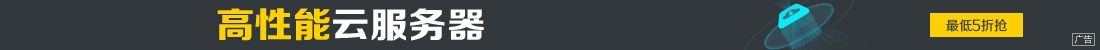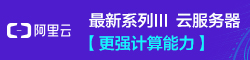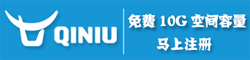# 浅谈Python数据类型判断及列表脚本操作

••TypeError: unorderable types: NoneType() < int()

var=' ' 或者 var=0

import types

type(x) is types.IntType

type(x) is types.StringType

if type(x) is types.IntType:
var1<var2a=[]
b=[1,2,3,4,5,6,7,8,9,0]
a.append(b[i:j]+b[j+1:k]+[b[k+1]-m])a.append(b[i]+b[j])